The Formula node allows you to create a formula that can be applied to several nodes at the same time. For example, you create a Formula node and define two outputs - Width and Height - and link them to several generators. When you modify the formula, all linked generators use the new sizes, for example 2048 instead of 1024.

This node can have 0 to 10 input connectors and 1 and 10 output connectors. Both the input and output connector formula results are numbers.

To add a Formula node, right click in the Graph Editor and select Create Node  > Calculation  > Formula.Double click on the node to open its parameters: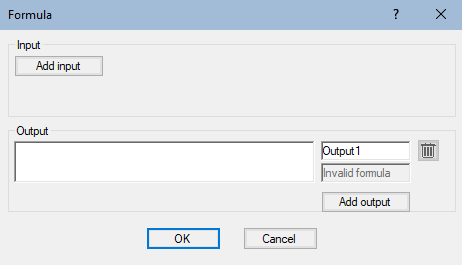Editing a Formula node

The parameters window allows you to add connectors and define formulas.

• Click Add input to add an input connector. Click again to add another, and so on. 0 to 10 input connectors are permitted.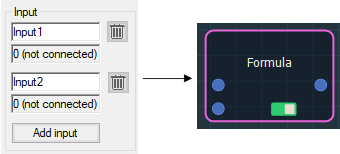The input connectors must link to other calculation nodes

• Click in the Input text field to change the input connector name.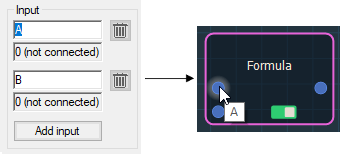• Delete an input connector by clicking on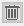.
• Click in the Output text field to change the output connector name.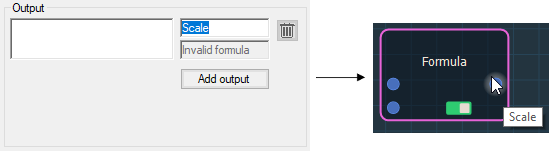• Click Add output to add an output connector. Click again to add another, and so on. 1 to 10 output connectors are permitted.
• For each output, write a formula that represents the value of the output connector. A formula can be a simple number.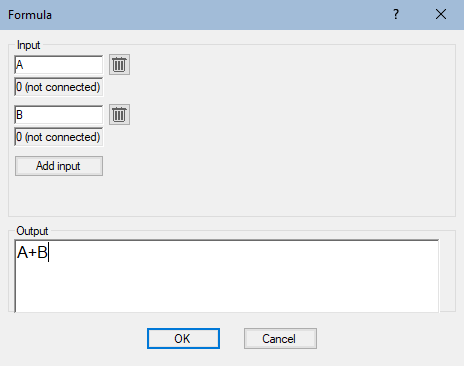• Delete an output connector by clicking on.

When a node is linked to the Formula node, the corresponding value in the node's properties is no longer editable. For example, if the Formula node is linked to the Coefficient of the first terrain, this parameter is grayed out in the Composition node's parameters.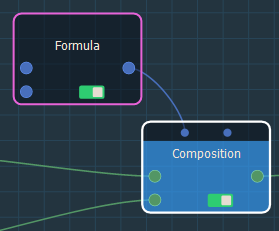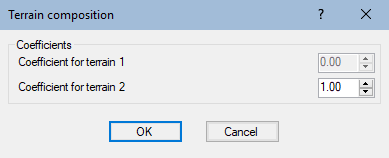Example

In the example below, the Equations formula node has two input connectors, X and Y, which take the values 400 and 150 defined by the X and Y formula nodes.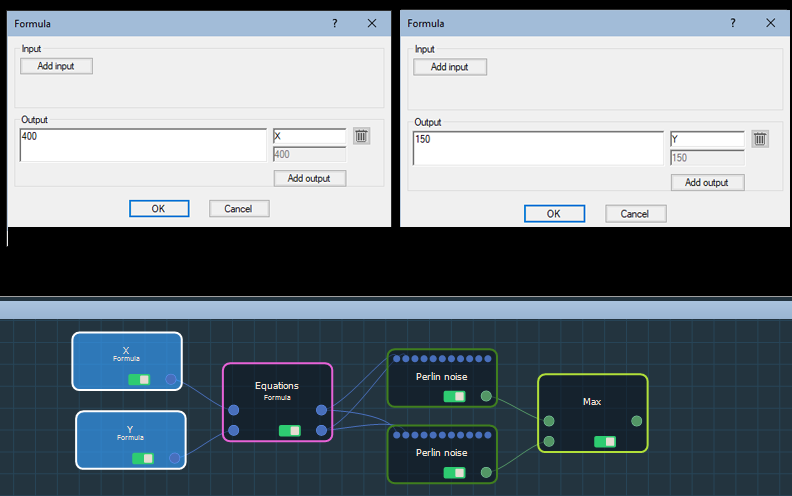The Equations node output connectors use these X and Y values in two formulas to define the width and height of two Perlin noise generators.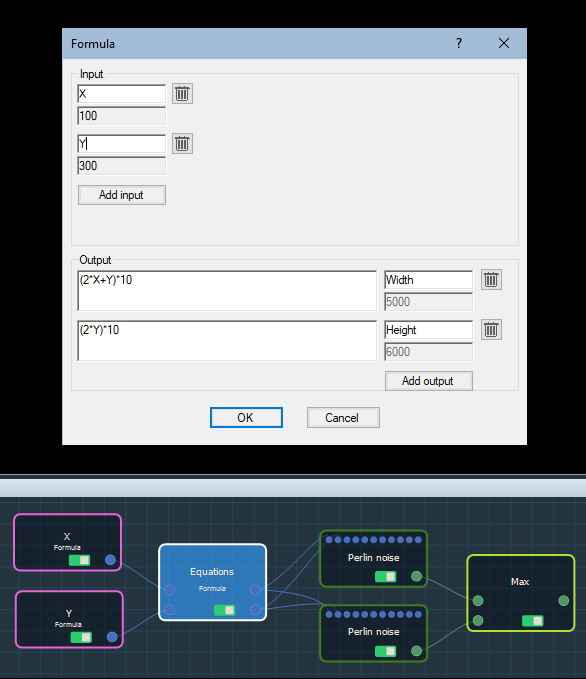The Width and Height parameters of both Perlin noise node parameters are non-editable because they are defined by the formula.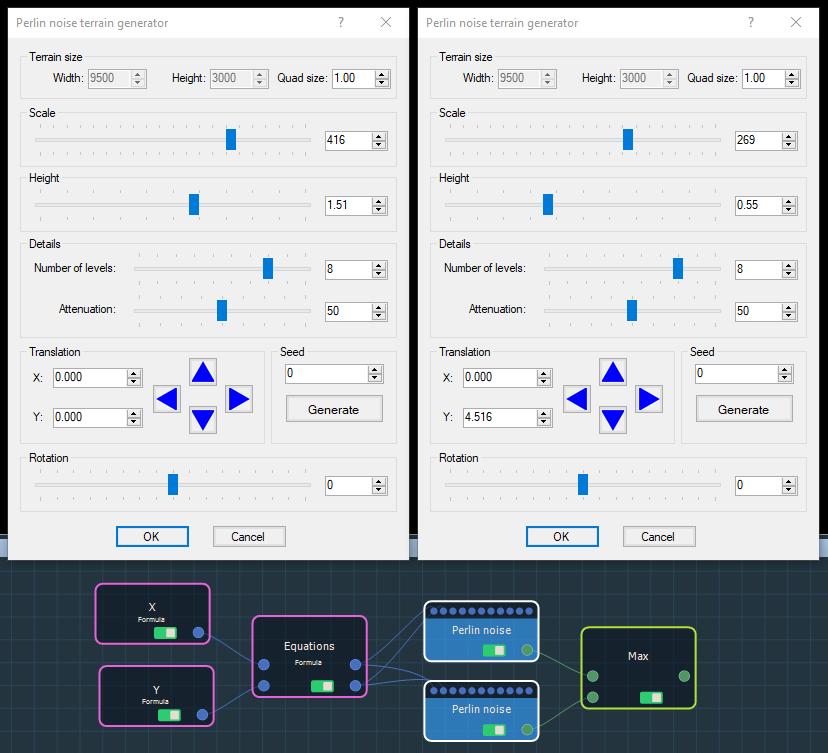The generators are combined using a Max node, where the value of the output terrain is the maximum value of both input terrains. Here is the result: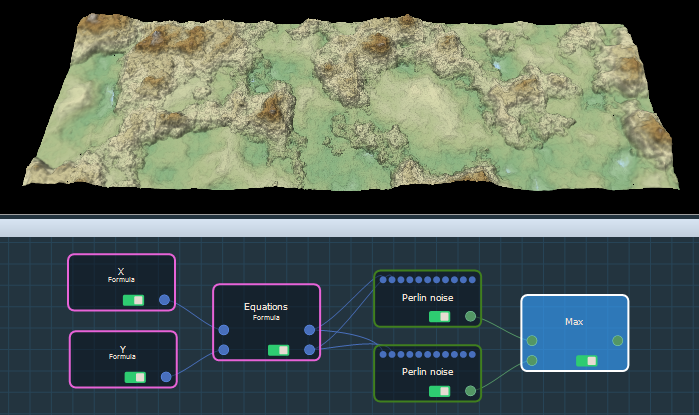Functions

 ABS(param) Returns the absolute value of the parameter. Example: ABS (-3) returns 3. SIGN(param) Returns 1 if param is strictly positive, -1 if param is strictly negative, 0 if param is null. MIN(param1; param2) Returns the minimum of a list of values. This function can have between 2 and 10 parameters separated by semicolons. MAX(param1; param2) Returns the maximum of a list of values. This function can have between 2 and 10 parameters separated by semicolons. AVERAGE(param1; param2) Returns the average of a list of values. This function can have between 2 and 10 parameters separated by semicolons. CLAMP(param; min; max) If param is in the range [min; max], returns param. If param is smaller than min, returns min. If param is greater than max, returns max. Example: CLAMP (5; 10; 20) returns 10, CLAMP (15; 10; 20) returns 15 and CLAMP (25; 10; 20) returns 20. SQR(param) Returns the square of param, i.e. param * param. SQRT(param) Returns the square root of param. SIN(angle) Returns the sine of angle expressed in radians. COS(angle) Returns the cosine of angle expressed in radians. TAN(angle) Returns the tangent of angle expressed in radians. LOG(param) Returns the natural logarithm of param: ln (param). LOG10(param) Returns the decimal logarithm of param: log10 (param) = ln (param) / ln (10). LOG2(param) Returns the binary logarithm of param: log2 (param) = ln (param) / ln (2). EXP(param) Returns the exponential of param: eparam. POW(param1; param2) Returns param1 to the param2 power: param1param2. ROUND(param) Rounds the value to the nearest integer: ROUND (1.8) returns 2, ROUND (1.2) returns 1. TRUNC(param) Returns the integer value obtained by removing the digits after the comma: TRUNC (1.2) returns 1, TRUNC (-1.2) returns -1. FLOOR(param) Returns the largest integer value less than equal to param: FLOOR (1.2) returns 1, FLOOR (-1.2) returns -2. CEILING(param) Returns the smallest integer value greater than or equal to param: CEILING (1.2) returns 2, CEILING (-1.2) returns -1. IF(test; param1; param2) Returns param1 if the test is true, param2 if the test is false. 'test' can use the following operators: <=,> =, >, <,>, =. Example: IF (A < 4; 10; 20) returns 10 if A is strictly less than 4, and 20 otherwise.